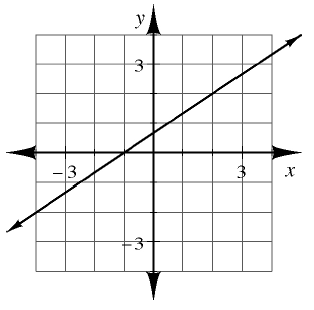### Home > CAAC > Chapter 6 > Lesson 6.3.1 > Problem6-105

6-105.

Multiple Choice: Martha’s equation has the graph shown at right. Which of these are solutions to Martha’s equation? (Remember that more than one answer may be correct.)1. $\quad ( - 4 , - 2 )$

1. $( - 1,0 )$

1. $x = 0 \text { and } y = 1$

1. $x = 2 \text { and } y = 2$

Which of the given ordered pairs is on the line?